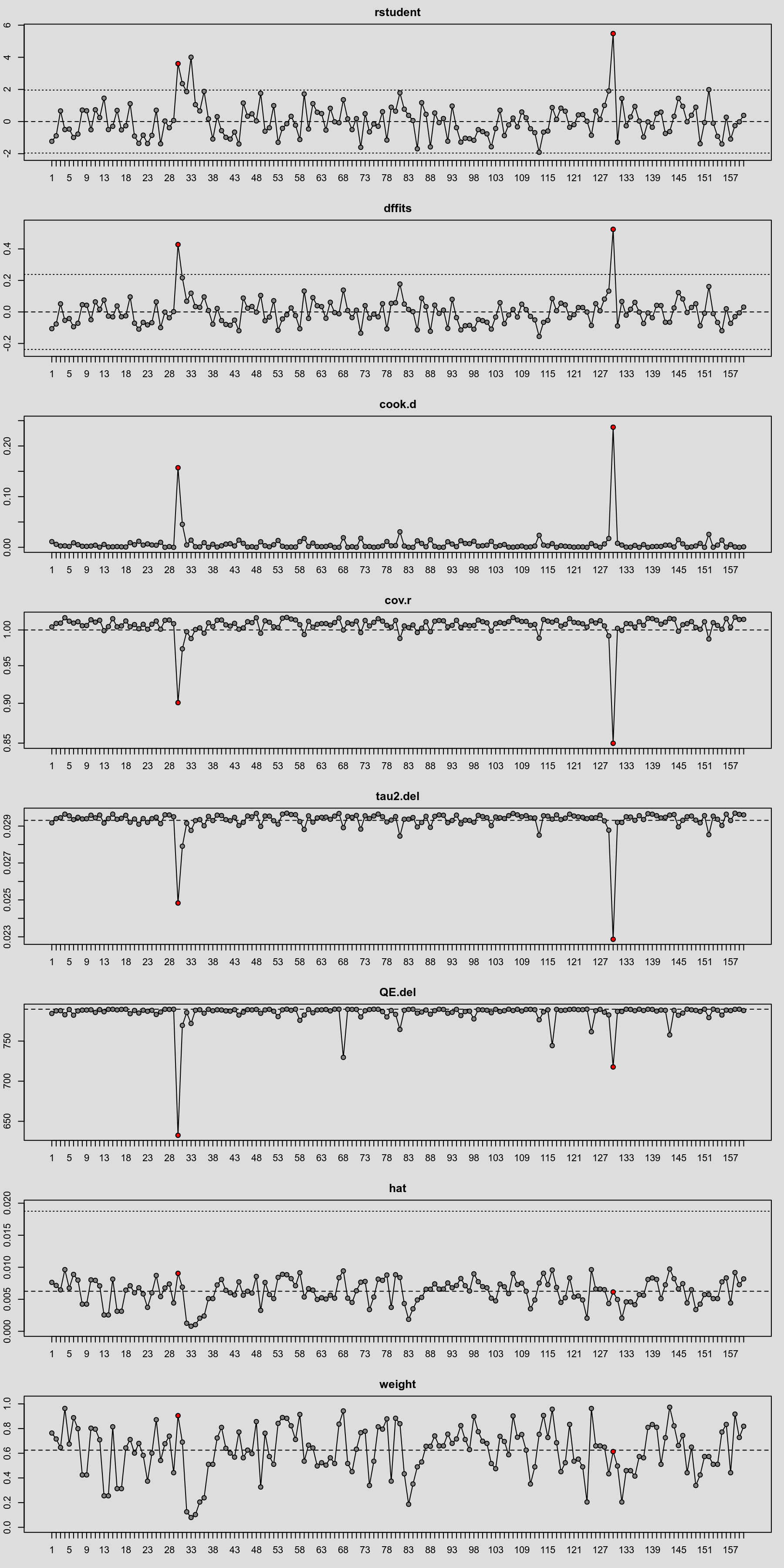#The metafor Package

A Meta-Analysis Package for R

### Site Tools

plots:plot_of_influence_diagnostics

## Plot of Influence Diagnostics

### Description

A variety of outlier and influential case diagnostics can be computed when conducting a meta-analysis (Viechtbauer & Cheung, 2010). The figure below shows a plot of the (1) externally standardized residuals, (2) DFFITS values, (3) Cook's distances, (4) covariance ratios, (5) leave-one-out estimates of the amount of heterogeneity, (6) leave-one-out values of the test statistics for heterogeneity, (7) hat values, and (8) weights. Three studies have rather large residuals and may be considered outliers. Only two of those studies actually have a strong influence on the results (as reflected, for example, in their Cook's distances). Removal of these studies would reduce the amount of heterogeneity quite a bit and increase the precision of the estimated average outcome (i.e., r-to-z transformed correlation) from the random-effects model. However, instead of just removing those studies, one should examine them in detail to determine what the reason may be for their unusual results. Outliers and influential cases can actually reveal patterns that may lead to new insights about study characteristics that could be acting as potential moderators (Light & Pillemer, 1984).

### Plot### Code

library(metafor)

### fit random-effects model with r-to-z transformed correlations
res <- rma(ri=ri, ni=ni, measure="ZCOR", data=dat.mcdaniel1994)

### calculate influence diagnostics
inf <- influence(res)

### plot the influence diagnostics
plot(inf, layout=c(8,1))

Light R. J., & Pillemer D. B. (1984). Summing up: The science of reviewing research. Cambridge, MA: Harvard University Press.

Viechtbauer, W., & Cheung, M. W.-L. (2010). Outlier and influence diagnostics for meta-analysis. Research Synthesis Methods, 1(2), 112–125.